### Lines and Angles - Worksheets

CBSE Worksheet-01
CLASS – V Mathematics –Complementary Angles

Identify the missing angle in each set of complementary angles.

 1.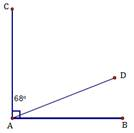2.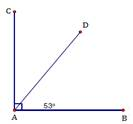3.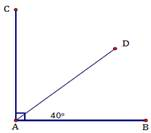4.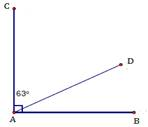5.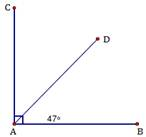6.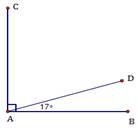7.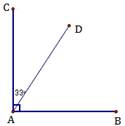8.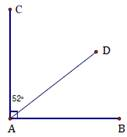9.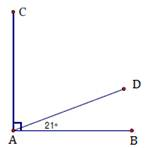10.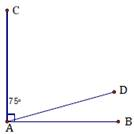11.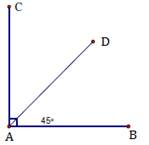12.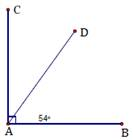CBSE Worksheet-01
CLASS – V Mathematics –Complementary Angles
Answers

 1.∠CAD=68o∠A=90o(Right Angle)∠DAB=900−68o=22o 2.∠BAD=53o∠A=90o(Right Angle)∠CAD=90o−53o=37o 3.∠BAD=40o∠A=90o(Right Angle)∠CAD=90o−40o=50o 4.∠CAD=63o∠A=90o(Right Angle)∠DAB=90o−63o=27o 5.∠BAD=47o∠A=90o(Right Angle)∠CAD=90o−47o=43o 6.∠BAD=17o∠A=90o(Right Angle)∠CAD=90o−17o=73o 7.∠CAD=33o∠A=90o(Right Angle)∠DAB=90o−33o=57o 8.∠CAD=52o∠A=90o(Right Angle)∠DAB=90o−52o=38o 9.∠BAD=21o∠A=90o(Right Angle)∠CAD=90o−21o=69o 10.∠CAD=75o∠A=90o(Right Angle)∠BAD=90o−75o=15o 11.∠BAD=45o∠A=90o(Right Angle)∠CAD=90o−45o=45o 12.∠BAD=54o∠A=90o(Right Angle)∠CAD=90o−54o=36o